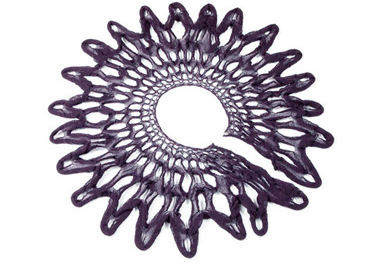list
Project name Fibonacci Scarf Diana Eng http://www.dianaeng.com/ fibonacci, scarf, knitting, patterns,This scarf is knit with the Fibonacci number pattern. Famous in the mathematics world, the Fibonacci number pattern is created by adding a number to the previous number: 0,1, 1, 2, 3, 5, 8, 13, 21, 34… (2 comes from adding 1 + 1, 3 comes from adding 2 + 1). This is no ordinary number sequence. The Fibonacci number patterns are found in nature in the seed placement of a sunflower, the pattern on a pine cone, and the uncurling of a fern. The number pattern creates a golden spiral and is used in financial predictions and for computer algorithms. This scarf is knit with the Fibonacci numbers in order: 1, 1, 2, 3, 5, 8, 13, and 21. As the scarf is knit, the new number of stitches is added to the previous number of stitches to get the next number of stitches. Thus the knitting method is also influence by the Fibonacci formula.